# HSPT Math : Arithmetic

## Example Questions

1 2 54 55 56 57 58 59 60 62 Next →

### Example Question #611 : Arithmetic

What is the sale price of a television if it is% off the original price of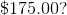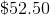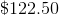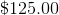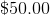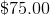Explanation:

First, multiply: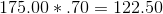Then subtract that amount from the original price: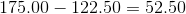Answer: The sale price is.

### Example Question #611 : Arithmetic

24 is what percent of 96?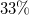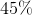Explanation:

Translate the sentence into an equation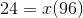Isolateand you get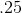.

Remember that the question is asking for a percentage, so convertinto.

1 2 54 55 56 57 58 59 60 62 Next →

### All HSPT Math Resources